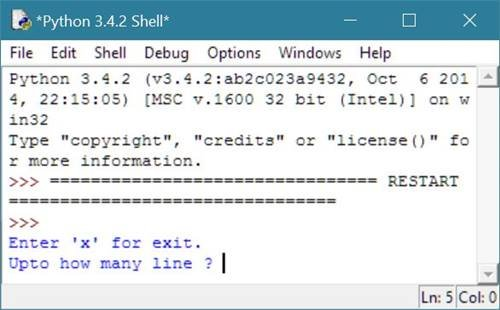# Python Program to Print Floyd's Triangle

## Print Floyd's Triangle in Python

To print Floyd's triangle in python, you have to ask from user to enter the number of rows or line upto which he/she want to print the desired Floyd triangle as shown in the program given below.

## Python Programming Code to Print Floyd's Triangle

Following python program ask from user to enter total number of line to print Floyd triangle expanded upto the given number of line or rows:

```# Python Program - Print Floyd Triangle

print("Enter 'x' for exit.")
ran = input("Upto how many line ? ")
if ran == 'x':
exit();
else:
rang = int(ran)
k = 1
for i in range(1, rang+1):
for j in range(1, i+1):
print(k, end=" ")
k = k + 1
print()
```

Here is the sample run of the above python program to illustrates how to print Floyd triangle:Now enter total number of line to print floyd triangle upto that number of line say 5 and press enter key:Here is the same program on python shell:### Same Program in Other Languages

You may also like to learn or practice the same program in other popular programming languages:

Tools
Calculator